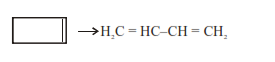# Gaseous cyclobutene isomerizes to butadiene in a first order process which has a 'k' value`
Question:

Gaseous cyclobutene isomerizes to butadiene in a first order process which has a 'k' value of $3.3 \times 10^{-4} \mathrm{~s}^{-1}$ at $153^{\circ} \mathrm{C}$. The time in minutes it takes for the isomerization to proceed $40 \%$ to completion at this temperature is _____. (Rounded off to the nearest integer)

Solution:$\mathrm{Kt}=\ln \frac{[\mathrm{A}]_{0}}{[\mathrm{~A}]_{\mathrm{t}}}$

$3.3 \times 10^{-4} \times \mathrm{t}=\ell \mathrm{n}\left(\frac{100}{60}\right)$

$\mathrm{t}=1547.956 \mathrm{sec}$

$\mathrm{t}=25.799 \mathrm{~min}$

26 min